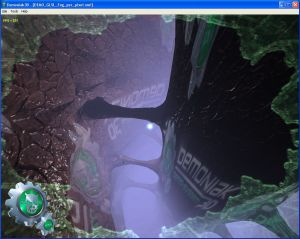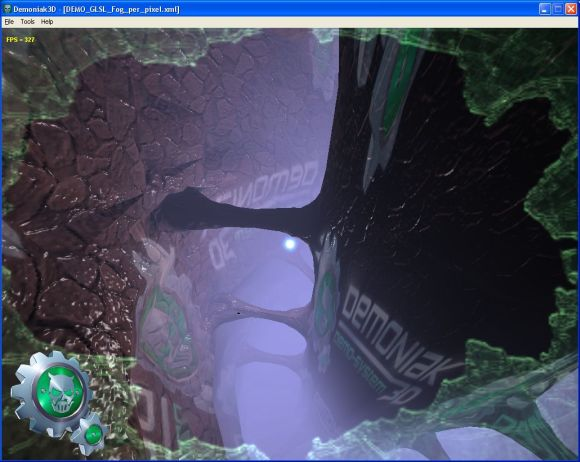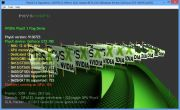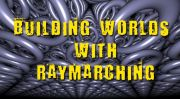Home Utilities Tutorials 3D Demos Graphics Cards Forums About

 GeeXLab
Current version: 0.45.1
>GeeXLab homepage

 FurMark
Current version: 1.30.0
>FurMark homepage

 GPU Caps Viewer
Current version: 1.55.0.0
>GPU Caps Viewer homepage

 GPU Shark
Current version: 0.26.0.0
>GPU Shark homepage

 Blogs
>JeGX's HackLab

 Geeks3D's Articles
>GPU Memory Speed Demystified

>How to Get your Multi-core CPU Busy at 100%

>How To Make a VGA Dummy Plug

>Night Vision Post Processing Filter

 PhysX FluidMark
Current version: 1.5.4
>FluidMark homepage

 TessMark
Current version: 0.3.0
>TessMark homepage

Current version: 0.3.0

 Demoniak3D
Current Version: 1.23.0
>Demoniak3D
>Libraries and Plugins
>Demos
>Codes SamplesFog in GLSL By Jerome 'JeGX' Guinot - jegx_AT_ozone3d(dot)net Initial draft: December 21, 2007 [ Index ]�Next Page3 - Per Vertex Fog ComputationThe following code is the complete shader code, used in the Demoniak3D demo. This shader shows how to add fog to a bump mapping rendered scene . In this first shader, the fog factor is computed per-vertex . ```[Vertex_Shader] varying float atten; varying float fogFactor; varying vec3 lightVec, viewVec; attribute vec3 tangent; void main(void) { gl_Position = ftransform(); gl_TexCoord = gl_MultiTexCoord0.xy; vec3 n = normalize(gl_NormalMatrix * gl_Normal); vec3 t = normalize(gl_NormalMatrix * tangent); vec3 b = cross(n, t); vec3 vVertex = vec3(gl_ModelViewMatrix * gl_Vertex); vec3 vLVec = gl_LightSource.position.xyz - vVertex; atten = 1.0 / (1.0 + 0.00001 * dot(vLVec, vLVec)); vec3 vVec = -vVertex; viewVec.x = dot(vVec, t); viewVec.y = dot(vVec, b); viewVec.z = dot(vVec, n); lightVec.x = dot(vLVec, t); lightVec.y = dot(vLVec, b); lightVec.z = dot(vLVec, n); const float LOG2 = 1.442695; gl_FogFragCoord = length(vVertex); fogFactor = exp2( -gl_Fog.density * gl_Fog.density * gl_FogFragCoord * gl_FogFragCoord * LOG2 ); fogFactor = clamp(fogFactor, 0.0, 1.0); } [Pixel_Shader] varying float atten; varying float fogFactor; varying vec3 lightVec, viewVec; uniform sampler2D normalMap; uniform sampler2D colorMap; uniform sampler2D colorMap2; void main (void) { vec3 lVec = normalize(lightVec); vec3 vVec = normalize(viewVec); vec4 base = texture2D(colorMap, gl_TexCoord*2.0); vec3 bump = normalize(texture2D(normalMap, gl_TexCoord*2.0).xyz*2.0-1.0); vec4 base2 = texture2D(colorMap2, gl_TexCoord*4.0); float diffuse = max( dot(lVec, bump), 0.0 ); float specular = pow(clamp(dot(reflect(-vVec, bump), lVec), 0.0, 1.0), gl_FrontMaterial.shininess ); vec4 vAmbient = gl_FrontLightProduct.ambient * base + (base2*0.4); vec4 vDiffuse = gl_FrontLightProduct.diffuse * diffuse * base; vec4 vSpecular = gl_FrontLightProduct.specular * specular; vec4 finalColor = (vAmbient + vDiffuse + vSpecular) * atten; gl_FragColor = mix(gl_Fog.color, finalColor, fogFactor ); }```DEMO_GLSL_Fog_per_vertex.xml At the shader vertex level, the interesting part of code is the following: ```const float LOG2 = 1.442695; gl_FogFragCoord = length(vVertex); fogFactor = exp2( -gl_Fog.density * gl_Fog.density * gl_FogFragCoord * gl_FogFragCoord * LOG2 ); fogFactor = clamp(fogFactor, 0.0, 1.0);``` We implement here a GL_EXP2 type fog. Let's explain quickly the code. The used equation is: fogFactor = exp(-(density * z)2) The exponential function can be written by a power of 2 : exp(x) = 2(x/log(2)) 1/log(2) = 1.442695 exp(x) = 2(1.442695 * x) At GLSL level, there exists a function which permits to raise 2 to any x power: exp2. So our equation becomes : exp(x) = exp2(1.442695 * x) avec x = -(density * z)2 The final equation is: fogFactor = exp2(density2 * z2 * 1.442695) z is the distance between the camera and the currently processed vertex in the vertex pipeline. Thanks to the fact that in the vertex shader, we are working in the camera space, the distance is equal to length(vVertex) where vVertex is the vertex position in the camera's space . In the case when there is no pixel shader, we have to store this distance in a variable gl_FogFragCoord so that the fixed pixel treatment unit could process the fog. In our case, this update is not compulsory. We only do it formally. So the following code is perfectly valid: ```const float LOG2 = 1.442695; float z = length(vVertex); fogFactor = exp2( -gl_Fog.density * gl_Fog.density * z * z * LOG2 ); fogFactor = clamp(fogFactor, 0.0, 1.0);``` gl_Fog is an uniform pre-built GLSL variable with the following structure: ```struct gl_FogParameters { vec4 color; float density; float start; float end; float scale; }; uniform gl_FogParameters gl_Fog;``` The fields of this variable are initialized by OpenGL when we specify the fog's parameters . The start, end and scale fields are used with a GL_LINEAR fog type . For an exponential fog, only the density and color are used. At the GLSL level, there exists an uniform variable gl_FogCoord. This variable contains the camera-vertex distance value and this value is valid only if, in OpenGL, we have used the following line in the fog initialization : `glFogi(GL_FOG_COORD_SRC, GL_FOG_COORDINATE);` On its side, Demoniak3D initializes the fog with : `glFogi(GL_FOG_COORD_SRC, GL_FRAGMENT_DEPTH);` This means that gl_FogCoord is not initialized and that the distance computation, at the fixed pipeline level, is not done in each vertex but in each pixel. It's the aim of the second shader . At the pixel shader level, the use of the fog occurs in the last line : `gl_FragColor = mix(gl_Fog.color, finalColor, fogFactor );` This line permits to make a color interpolation between the fog's color (gl_Fog.color) and that of the fragment (finalColor) accordingly to the fog factor value . [ Index ]�Next Page

 GeeXLab demosGLSL - Mesh exploderPhysX 3 cloth demoNormal visualizer with GS